# Chapter 30 Sources of the magnetic field Force

• Slides: 29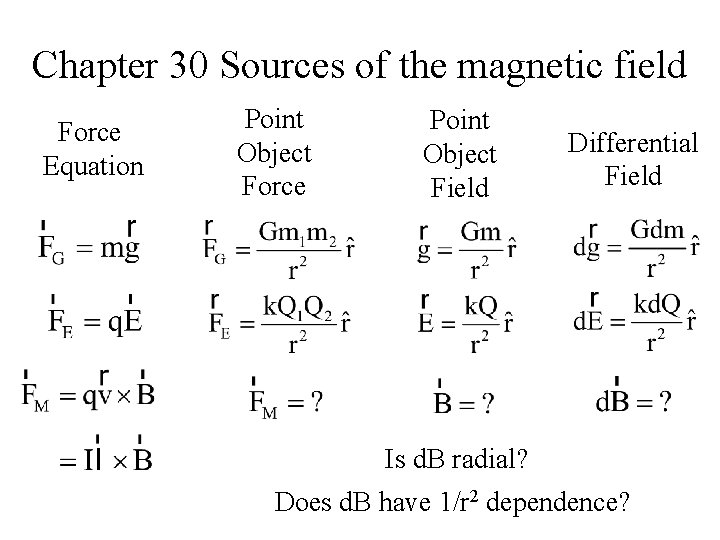Chapter 30 Sources of the magnetic field Force Equation Point Object Force Point Object Field Differential Field Is d. B radial? Does d. B have 1/r 2 dependence?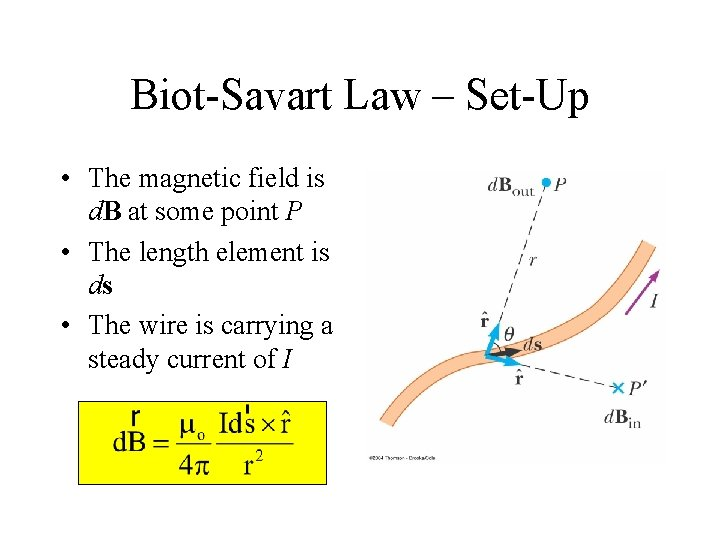Biot-Savart Law – Set-Up • The magnetic field is d. B at some point P • The length element is ds • The wire is carrying a steady current of I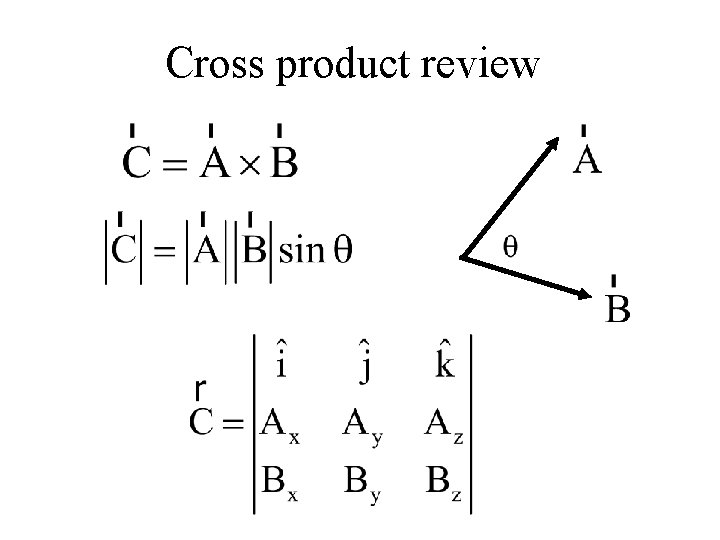Cross product review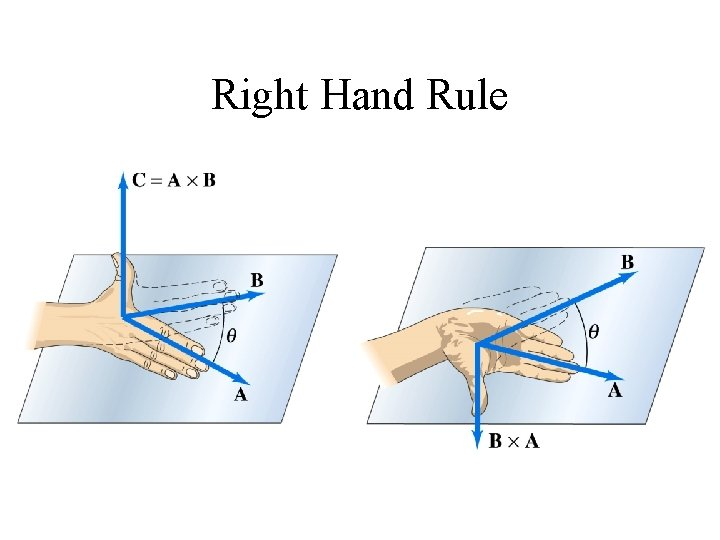Right Hand Rule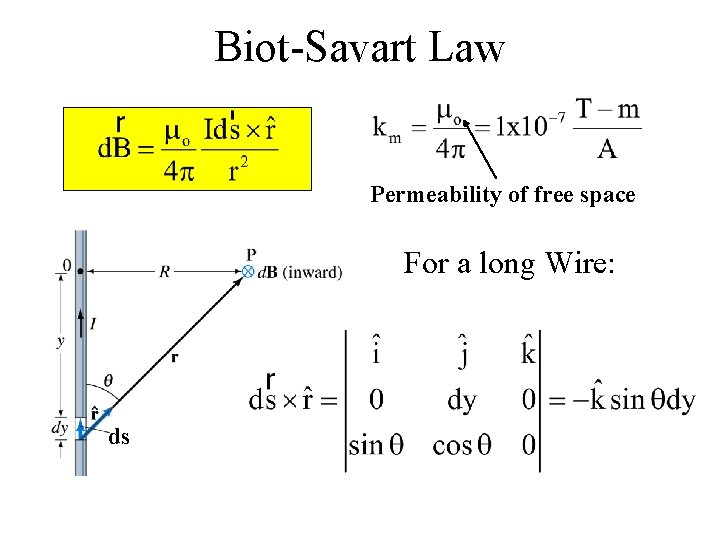Biot-Savart Law Permeability of free space For a long Wire: ds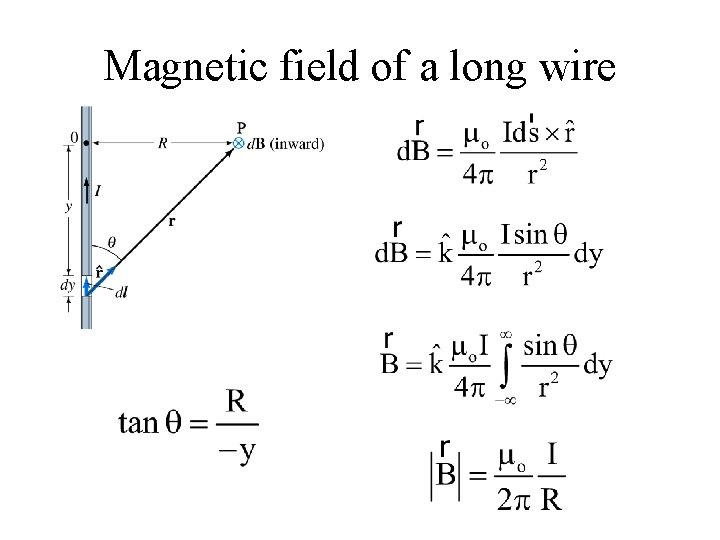Magnetic field of a long wireMagnetic field due to a straight wire Compare with:B for a Curved Wire Segment • Find the field at point O due to the wire segment • I and R are constants q will be in radians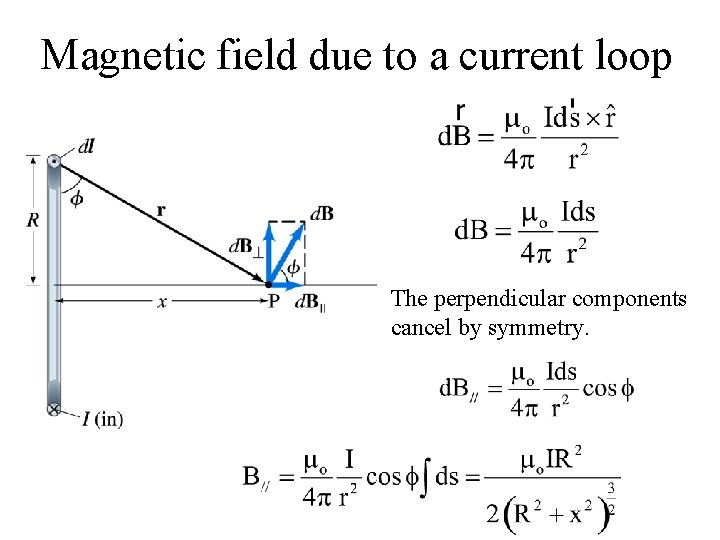Magnetic field due to a current loop The perpendicular components cancel by symmetry.Magnetic field due to a current loop At the center: Magnetic dipole moment vector for a single loop: When x>>R: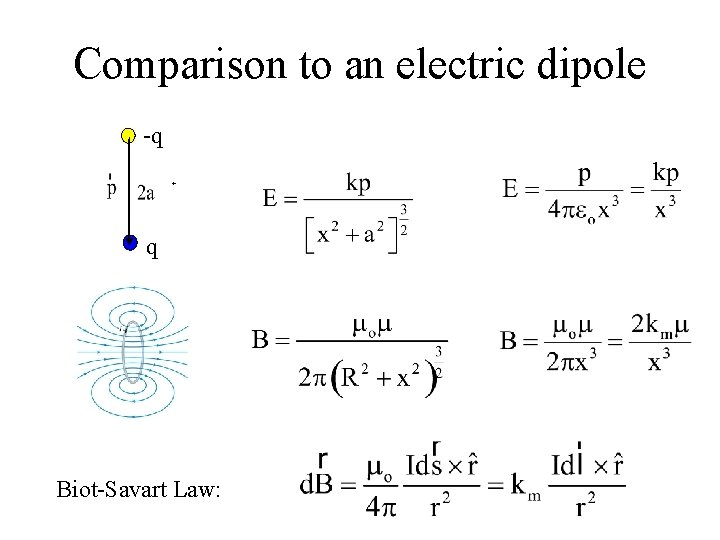Comparison to an electric dipole -q + q Biot-Savart Law: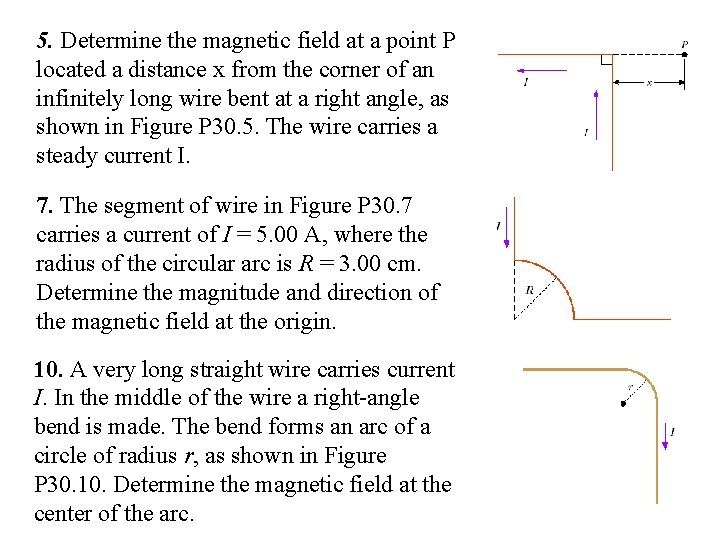5. Determine the magnetic field at a point P located a distance x from the corner of an infinitely long wire bent at a right angle, as shown in Figure P 30. 5. The wire carries a steady current I. 7. The segment of wire in Figure P 30. 7 carries a current of I = 5. 00 A, where the radius of the circular arc is R = 3. 00 cm. Determine the magnitude and direction of the magnetic field at the origin. 10. A very long straight wire carries current I. In the middle of the wire a right-angle bend is made. The bend forms an arc of a circle of radius r, as shown in Figure P 30. 10. Determine the magnetic field at the center of the arc.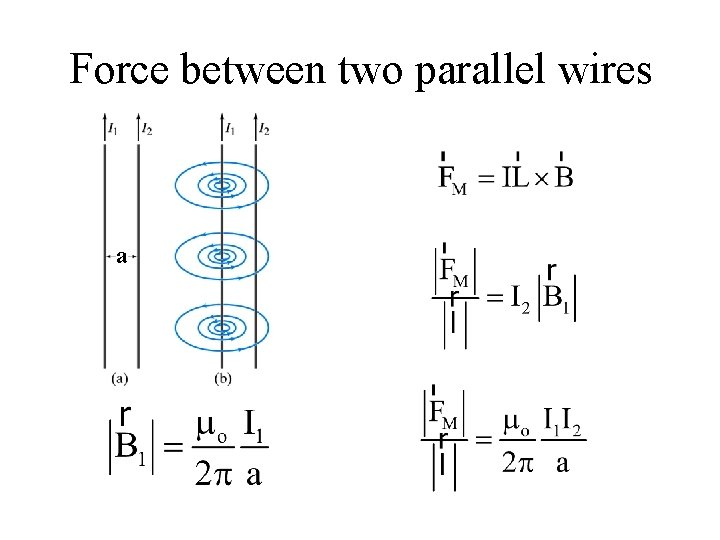Force between two parallel wires a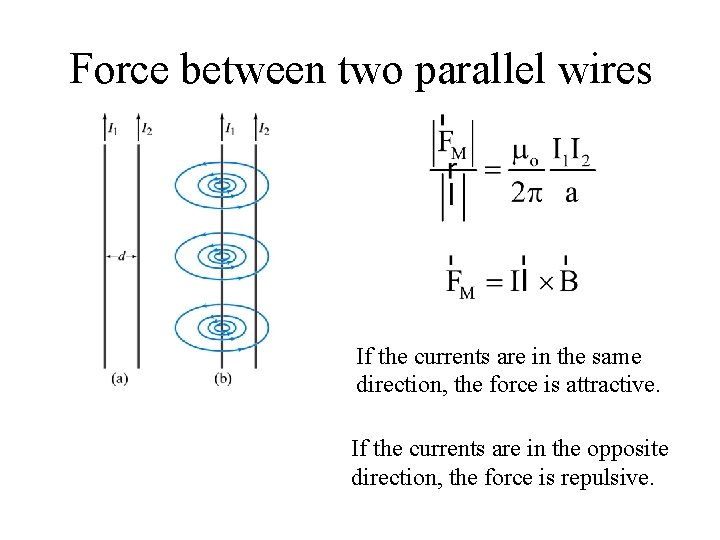Force between two parallel wires If the currents are in the same direction, the force is attractive. If the currents are in the opposite direction, the force is repulsive.Historical definition of the Ampere If 2 long parallel wires 1. 0 m apart have the same current in them and the force per unit length on each wire is 2. 0 x 10 -7 N/m, the current is 1. 0 Ampere Historical definition of the Coulomb If the current is 1. 0 Ampere, then 1. 0 Coulomb is the amount of charge passing through a cross section in 1 second.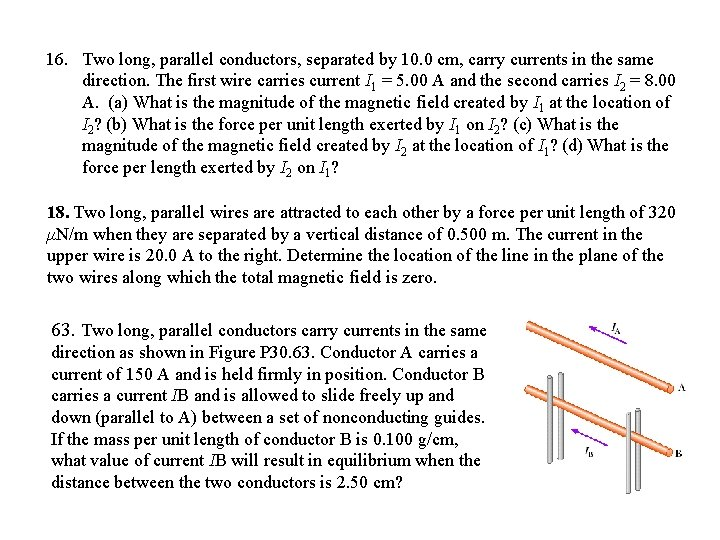16. Two long, parallel conductors, separated by 10. 0 cm, carry currents in the same direction. The first wire carries current I 1 = 5. 00 A and the second carries I 2 = 8. 00 A. (a) What is the magnitude of the magnetic field created by I 1 at the location of I 2? (b) What is the force per unit length exerted by I 1 on I 2? (c) What is the magnitude of the magnetic field created by I 2 at the location of I 1? (d) What is the force per length exerted by I 2 on I 1? 18. Two long, parallel wires are attracted to each other by a force per unit length of 320 μN/m when they are separated by a vertical distance of 0. 500 m. The current in the upper wire is 20. 0 A to the right. Determine the location of the line in the plane of the two wires along which the total magnetic field is zero. 63. Two long, parallel conductors carry currents in the same direction as shown in Figure P 30. 63. Conductor A carries a current of 150 A and is held firmly in position. Conductor B carries a current IB and is allowed to slide freely up and down (parallel to A) between a set of nonconducting guides. If the mass per unit length of conductor B is 0. 100 g/cm, what value of current IB will result in equilibrium when the distance between the two conductors is 2. 50 cm?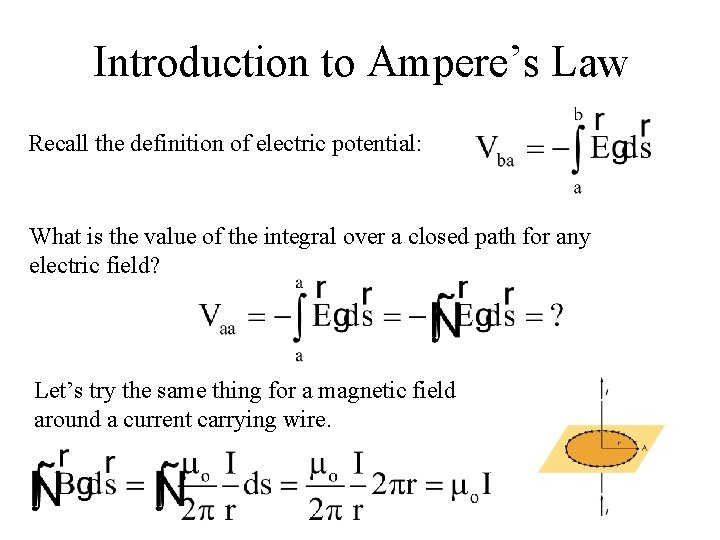Introduction to Ampere’s Law Recall the definition of electric potential: What is the value of the integral over a closed path for any electric field? Let’s try the same thing for a magnetic field around a current carrying wire.Ampere’s Law This result has been shown experimentally to be true in general • The integral is around any closed path • The current is that passing through the surface bounded by the path • Like Gauss’s Law, useful in finding fields for highly symmetric problems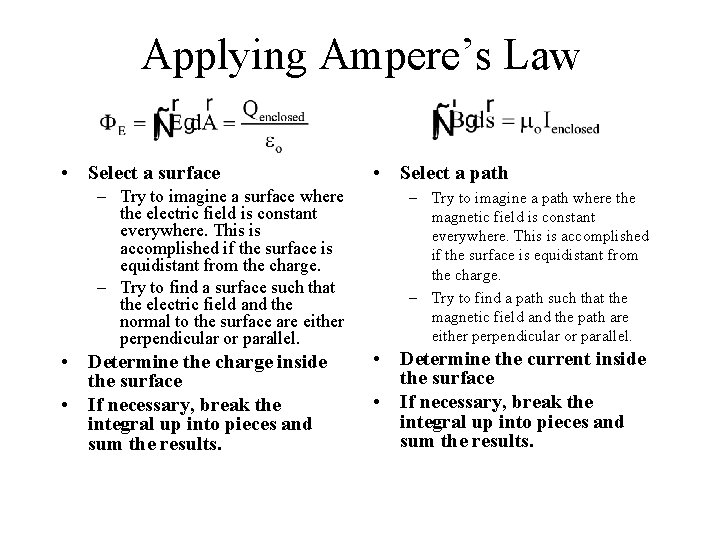Applying Ampere’s Law • Select a surface – Try to imagine a surface where the electric field is constant everywhere. This is accomplished if the surface is equidistant from the charge. – Try to find a surface such that the electric field and the normal to the surface are either perpendicular or parallel. • Determine the charge inside the surface • If necessary, break the integral up into pieces and sum the results. • Select a path – Try to imagine a path where the magnetic field is constant everywhere. This is accomplished if the surface is equidistant from the charge. – Try to find a path such that the magnetic field and the path are either perpendicular or parallel. • Determine the current inside the surface • If necessary, break the integral up into pieces and sum the results.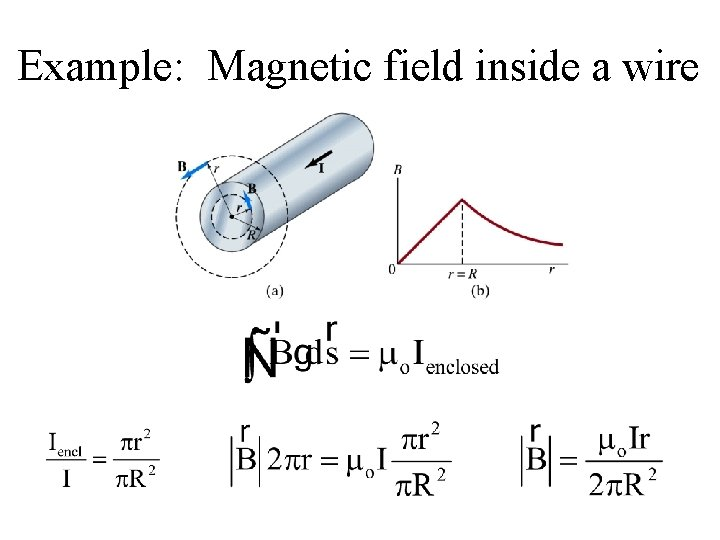Example: Magnetic field inside a wire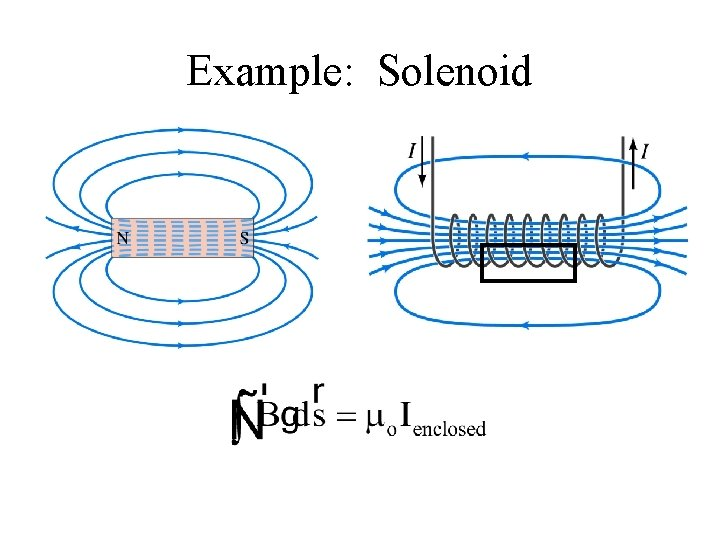Example: Solenoid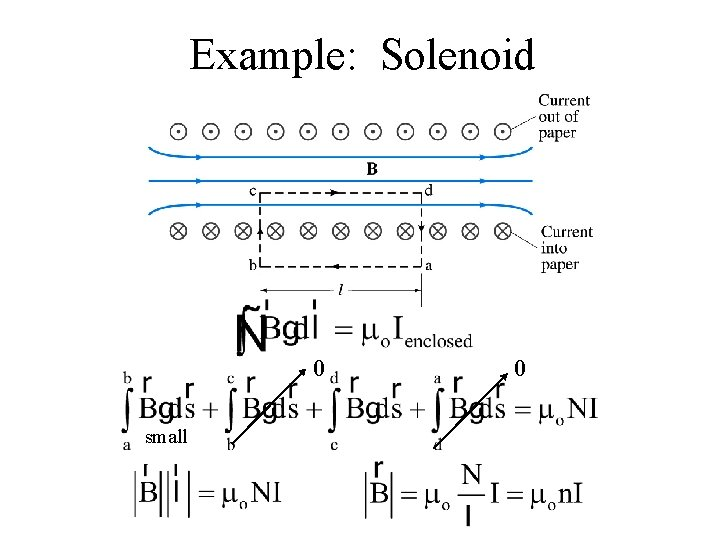Example: Solenoid 0 small 0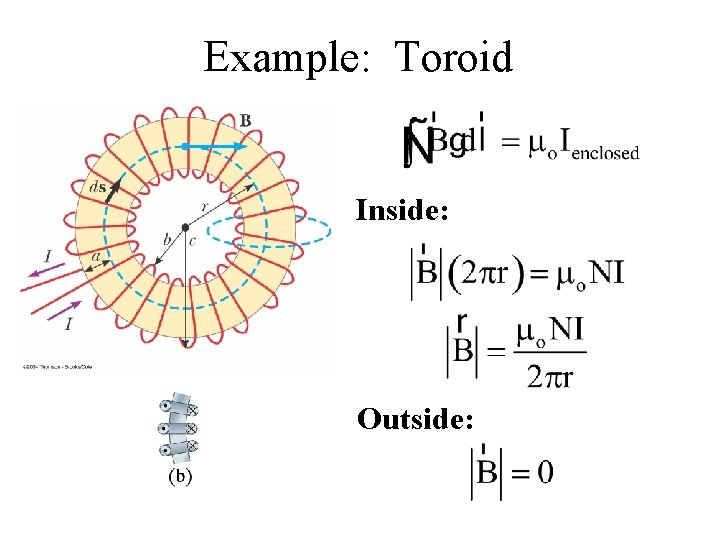Example: Toroid Inside: Outside:21. Four long, parallel conductors carry equal currents of I = 5. 00 A. Figure P 30. 21 is an end view of the conductors. The current direction is into the page at points A and B (indicated by the crosses) and out of the page at C and D (indicated by the dots). Calculate the magnitude and direction of the magnetic field at point P, located at the center of the square of edge length 0. 200 m. 29. A long cylindrical conductor of radius R carries a current I as shown in Figure P 30. 29. The current density J, however, is not uniform over the cross section of the conductor but is a function of the radius according to J = br, where b is a constant. Find an expression for the magnetic field B (a) at a distance r 1 < R and (b) at a distance r 2 > R, measured from the axis. 24. The magnetic field 40. 0 cm away from a long straight wire carrying current 2. 00 A is 1. 00 μT. (a) At what distance is it 0. 100 μT? (b) What If? At one instant, the two conductors in a long household extension cord carry equal 2. 00 -A currents in opposite directions. The two wires are 3. 00 mm apart. Find the magnetic field 40. 0 cm away from the middle of the straight cord, in the plane of the two wires. (c) At what distance is it one tenth as large? (d) The center wire in a coaxial cable carries current 2. 00 A in one direction and the sheath around it carries current 2. 00 A in the opposite direction. What magnetic field does the cable create at points outside?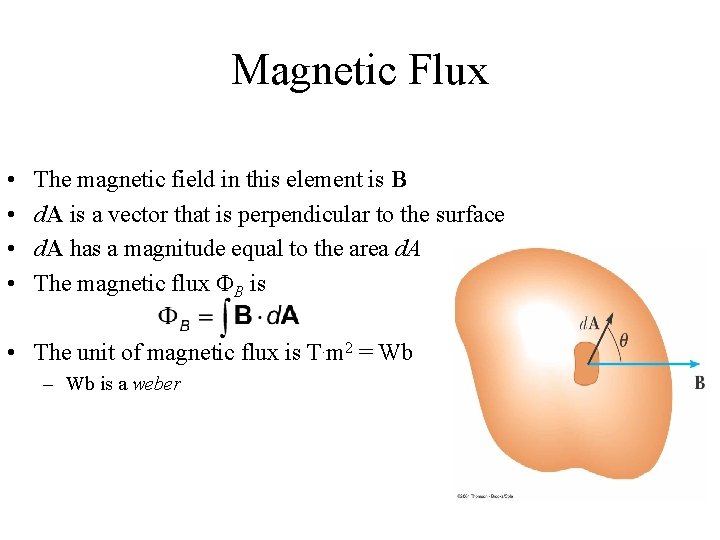Magnetic Flux • • The magnetic field in this element is B d. A is a vector that is perpendicular to the surface d. A has a magnitude equal to the area d. A The magnetic flux FB is • The unit of magnetic flux is T. m 2 = Wb – Wb is a weber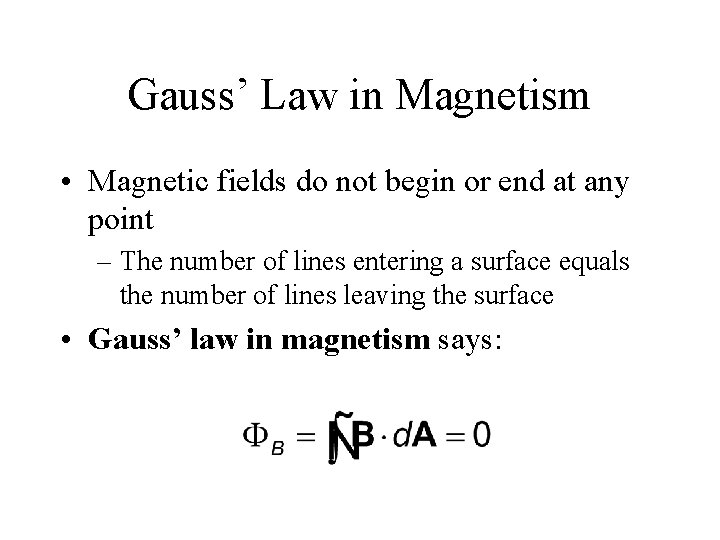Gauss’ Law in Magnetism • Magnetic fields do not begin or end at any point – The number of lines entering a surface equals the number of lines leaving the surface • Gauss’ law in magnetism says: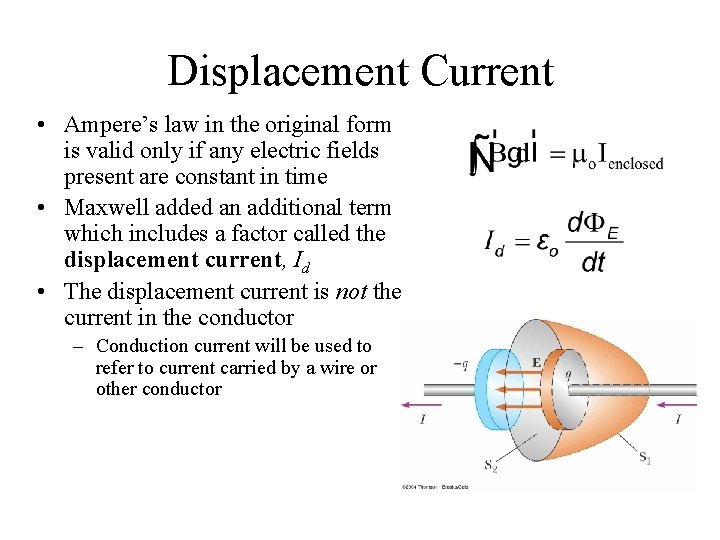Displacement Current • Ampere’s law in the original form is valid only if any electric fields present are constant in time • Maxwell added an additional term which includes a factor called the displacement current, Id • The displacement current is not the current in the conductor – Conduction current will be used to refer to current carried by a wire or other conductorAmpere’s Law – General Form • Also known as the Ampere-Maxwell law • Magnetic fields are produced both by conduction currents and by time-varying electric fieldsFerromagnetism • Domains • Curie Temperature • Electron orbits align with an external magnetic field